# Graphs and its Applications

Graphs in data structures are non-linear data structures made up of a finite number of nodes or vertices and the edges that connect them. Graph is an important topic when it comes to competitive examinations like GATE. You need to dive deep into its applications and types. The information contained in the notes will help you understand this topic in a better way.

## What is Graph?

A graph is a non-linear data structure that permits you to define connections between different types of data (nodes). Or we can say that a graph is a data structure that is built with the help of nodes or vertices and edges(arcs).

## Types of Graphs

Finite Graph: If a graph has a finite number of vertices and a finite number of edges, it is known as a finite graph.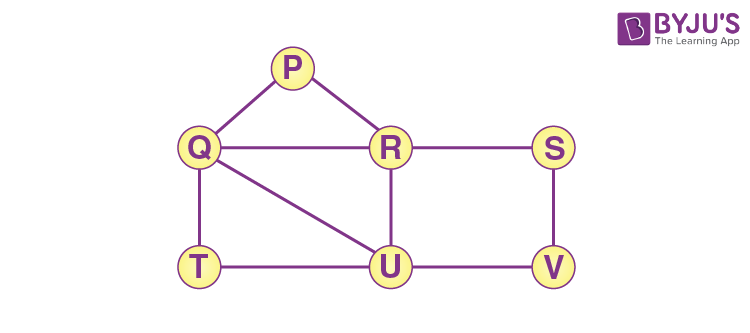Infinite Graph: If a graph has an infinite number of vertices and an infinite number of edges, it is known as an infinite graph.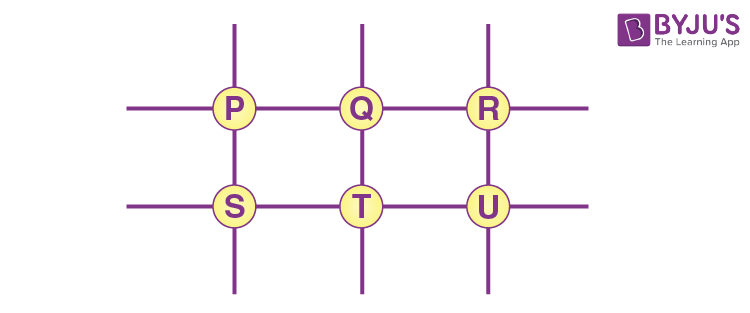Trivial Graph: If a finite graph has only a single vertex and no edge, it is known as a trivial graph.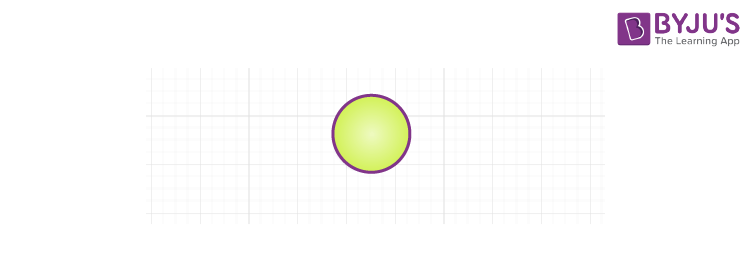Simple Graph: A graph that contains only one edge between the pair of vertices is known as a simple graph.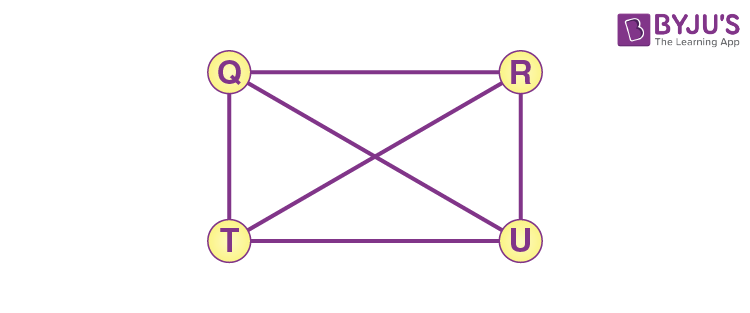Multi Graph: If there are multiple edges or points between a set of vertices in a graph, it is known as a multigraph.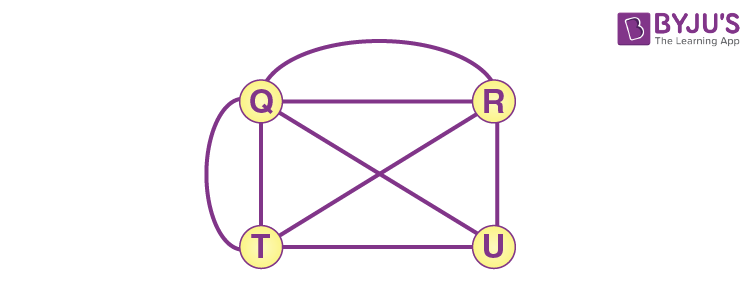Null Graph: A graph that does not include any edges in it is known as a null graph.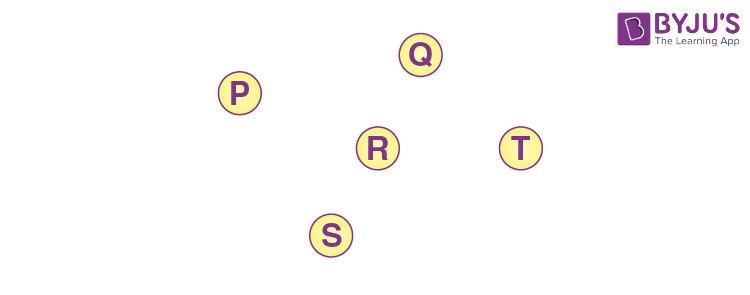Complete Graph: A complete graph is also known as a full graph because each vertex’s degree should be n-1.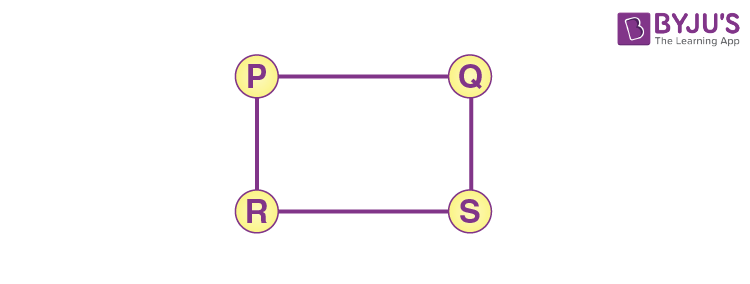Pseudo Graph: If a graph has a self-loop with other edges it is known as a pseudo graph.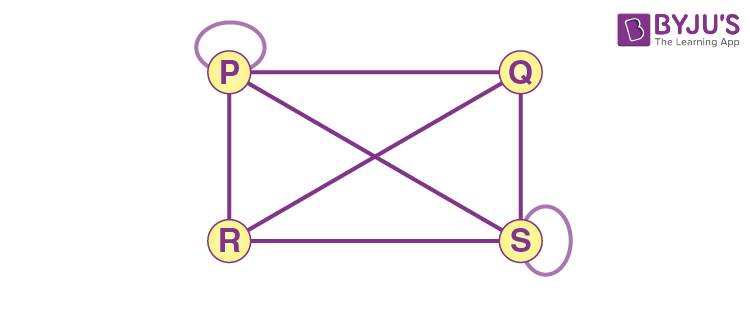Regular Graph: If each vertex has the same degree, it is known as a regular graph.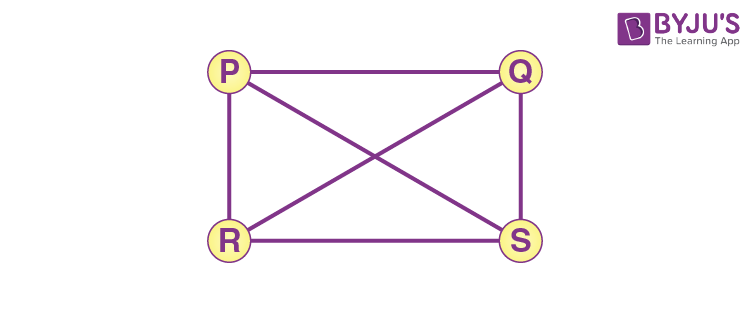Weighted Graph: A graph in which each edge has numbers or values is known as a weighted graph. It is a special type of graph.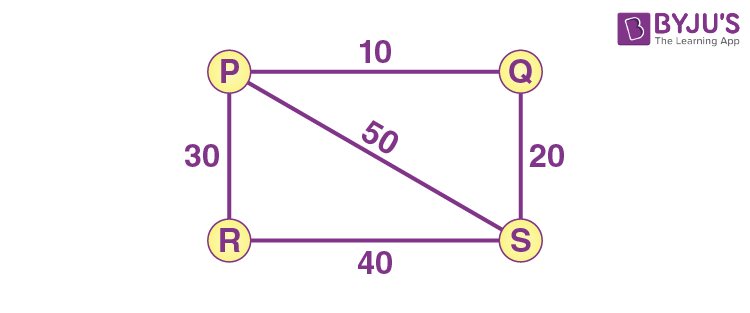Directed Graph: A directed graph, also referred to as a digraph, is a collection of vertices combined by edges, each with a direction.Undirected Graph: An undirected graph comprises a set of nodes and links connecting them. The order of the two connected vertices is irrelevant and has no direction. You can form an undirected graph with a finite number of vertices and edges.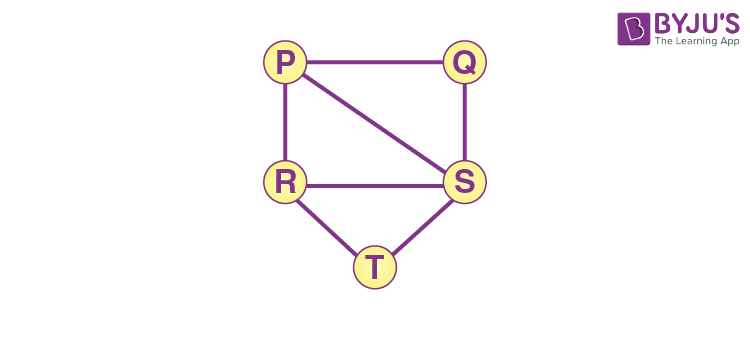Connected Graph: If there is a path between one vertex of a graph data structure and any other vertex, the graph is connected.### Graph Representation

There are two techniques to represent a graph:

In the adjacency matrix, each row and column define a vertex. It is a 2D array of V x V vertices.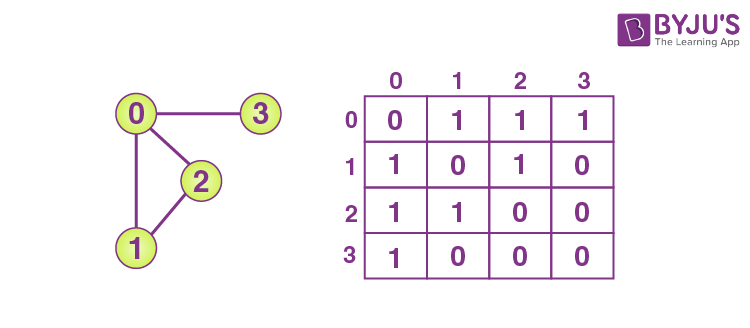The index of the array defines a vertex, and every component in its linked list describes the other vertices that form an edge with the vertex.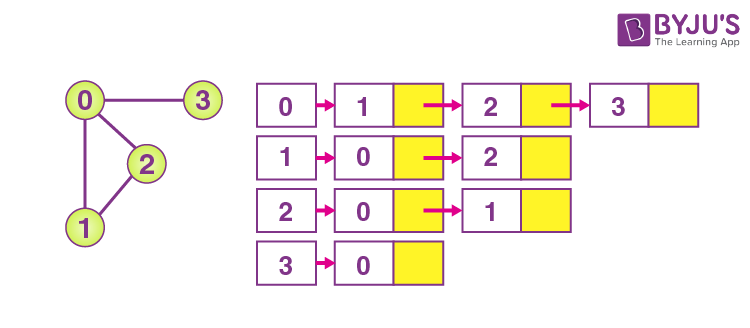Application of Graphs in Data Structures

Following are some applications of graphs in data structures:

• Graphs are utilised in computer science to describe the flow of analysis and computation.
• It is utilised to describe networks of communication.
• It is used to define data organisation.

## Practice Problem – Graph

Q. The Breadth First search (BFS) algorithm has been implemented using the queue data structure. Which one of the following is a possible order of visiting the nodes in the graph below?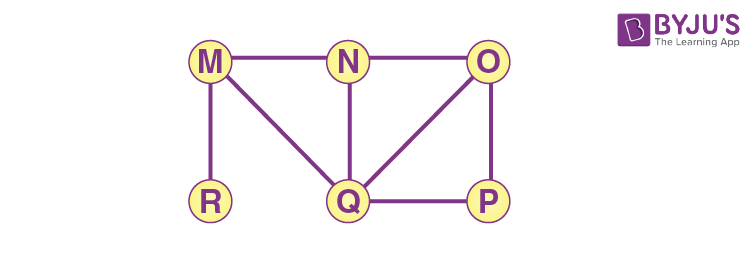• (A) MNOPQR
• (B) NQMPOR
• (C) QMNROP
• (D) POQNMR

Keep learning and stay tuned to get the latest updates on GATE Exam along with GATE Eligibility CriteriaGATE 2023GATE Admit CardGATE Syllabus for CSE (Computer Science Engineering)GATE CSE NotesGATE CSE Question Paper, and more.

Also Explore,